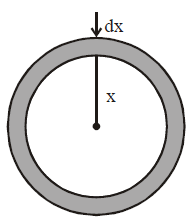# Q. To mop-clean a floor, a cleaning machine presses a circular mop of radius R vertically down with a total force F and rotates it with a constant angular speed about its axis. If the force F is distributed uniformly over the mop and if coefficient of friction between the mop and the floor is $\mu$, the torque, applied by the machine on the mop is :

JEE Main JEE Main 2019

Solution:

## Consider a strip of radius x & thickness dx, Torque due to friction on this strip. $\int d\tau =\int^{R}_{0} \frac{x\mu F.2\pi x dx }{\pi R^{2}}$ $\tau = \frac{ 2\mu F}{R^{2}} . \frac{R^{3}}{3}$ $\tau = \frac{2 \mu FR}{ 3}$ $\therefore$ correct answer is (1)You must select option to get answer and solution

## 1. The potential to which a conductor is raised, depends on ........

KCET 2005 Electrostatic Potential And Capacitance

## 2. The refractive index of a particular material is 1.67 for blue light, 1.65 for yellow light and 1.63 for red light. The dispersive power of the material is .........

KCET 2004 Electrostatic Potential And Capacitance

## 3. In a permanent magnet at room temperature

KCET 2019 Moving Charges And Magnetism

## 4. An antenna uses electromagnetic waves of frequency 5 MHz. For proper working, the size of the antenna should be

KCET 2019 Communication Systems

## 5. In the following circuit, what are P and Q?

KCET 2019 Semiconductor Electronics: Materials Devices And Simple Circuits

## 6. Two metal plates are separated by 2 cm. The potentials of the plates are − 10 V and + 30 V. The electric field between the two plates is

KCET 2019 Electrostatic Potential And Capacitance

KCET 2019 Nuclei

## 8. For a transistor amplifier, the voltage gain

KCET 2019 Semiconductor Electronics: Materials Devices And Simple Circuits

## 9. “Parsec” is the unit of

BHU 2007 Physical World, Units and Measurements

## 10. “Parsec” is the unit of

BHU 2007 Physical World, Units and Measurements